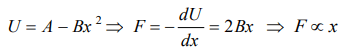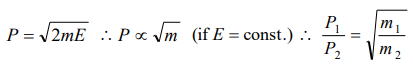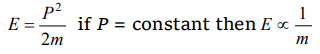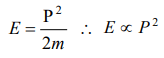## Work, Energy and Power Questions and Answers Part-7

1. The potential energy of a body is given by,$U=A-Bx^{2}$   (Where x is the displacement). The magnitude of force acting on the particle is
a) Constant
b) Proportional to x
c) Proportional to $x^{2}$
d) Inversely proportional to x

Explanation:2. The potential energy between two atoms in a molecule is given by $U\left(x\right)=\frac{a}{x^{12}}-\frac{b}{x^{6}}$    ; where a and b are positive constants and x is the distance between the atoms. The atom is in stable equilibrium when
a) $x = \sqrt{\frac{11a}{5b}}$
b) $x = \sqrt{\frac{a}{2b}}$
c) x = 0
d) $x = \sqrt{\frac{2a}{b}}$

Explanation:3. Which one of the following is not a conservative force
a) Gravitational force
b) Electrostatic force between two charges
c) Magnetic force between two magnetic dipoles
d) Frictional force

Explanation: Friction is a non-conservative force

4. Two bodies of masses $m_{1}$ and $m_{2}$ have equal kinetic energies. If $p_{1}$ and $p_{2}$ are their respective momentum, then ratio $p_{1}:p_{2}$ is equal to
a) $m_{1}:m_{2}$
b) $m_{2}:m_{1}$
c) $\sqrt{m_{1}}:\sqrt{m_{2}}$
d) $m_1^2:m_2^2$

Explanation:5. Work done in raising a box depends on
a) How fast it is raised
b) The strength of the man
c) The height by which it is raised
d) None of the above

Explanation: Work in raising a box = (weight of the box) × (height by which it is raised)

6. A light and a heavy body have equal momenta. Which one has greater K.E
a) The light body
b) The heavy body
c) The K.E. are equal
d) Data is incomplete

Explanation:7. A body at rest may have,
a) Energy
b) Momentum
c) Speed
d) Velocity

Explanation: Body at rest may possess potential energy

8. The kinetic energy possessed by a body of mass m moving with a velocity v is equal to$\frac{1}{2}mv^{2}$  , provided
a) The body moves with velocities comparable to that of light
b) The body moves with velocities negligible compared to the speed of light
c) The body moves with velocities greater than that of light
d) None of the above statement is correcst

Explanation: Due to theory of relativity

9. If the momentum of a body is increased n times, its kinetic energy increases
a) n times
b) 2n times
c) $\sqrt{n}$  times
d) $n^{2}$  timesi.e. if P is increased n times then E will increase n2 times